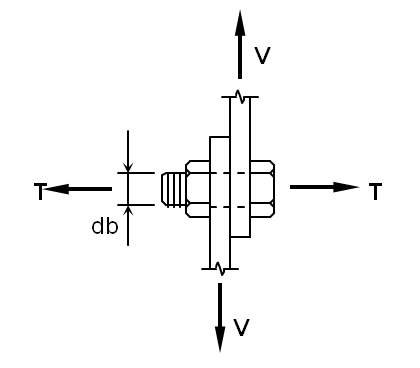Bolt Single and Double Shear and Tension Stress Analysis Calculator per AISC

Strength of Materials

This calculator will deteremine bolt stress in tension and shear per AISC 9th Edition Manual (ASD)

 BOLT STRESS ANALYSIS For High-Strength Bolts Subject to Tension and/or Shear?? Per AISC 9th Edition Manual (ASD)??Input Data: Tension Force/Bolt, T = kips/bolt Shear Force/Bolt, V = kips/bolt Bolt Diameter, db = 0.750 0.875 1.000 1.125 1.250 1.375 1.500 in. ASTM Bolt Desig. = A325 A490 Bolt Type (N, X, or SC) = N X SC Bolt Hole Type = Standard Oversized Single or Double Shear? Single Double No. of Loading Cycles = (for 25 years) Results: Ab = in.2 Ab = p*db2/4 Tb = ksi Bolt Tension: ft = ksi ft = T/Ab ksi Use: Ft = ksi T = kips/bolt B = kips/bolt Bolt Shear: fv = ksi fv = V/Ab Fv = ksi Shear Fact. = V = kips/bolt Vb = kips/bolt Vb = Fv*Ab*(SF) SR = SR = SUMMARY OF CHECKS: Row No.: Results: Stress Ratio: Bolt Tension: 31 Bolt Shear: 39Membership Register | LoginHomeEngineering Book StoreEngineering ForumExcel App. DownloadsOnline Books & ManualsEngineering NewsEngineering VideosEngineering CalculatorsEngineering ToolboxGD&T Training Geometric Dimensioning TolerancingDFM DFA TrainingTraining Online EngineeringAdvertising CenterCopyright Notice